# Calculate Equivalent Impedance in AC Circuits

   

Examples on how to use the rules of impedances connected in series and parallel to calculate equivalent impedances in various AC circuits and present them as complex numbers in standard, complex and polar forms. Detailed solutions to the examples are also presented.

## Examples with Solutions

Example 1
Find the equivalent impedance between points A and B in the circuit given below and write it in exponential and polar form. .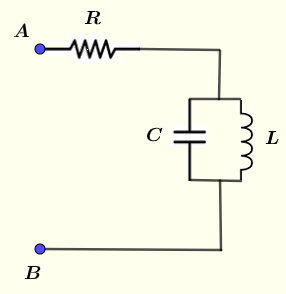Solution to Example 1
Let $Z_1$ be the impedance of resistor R and hence $Z_1 = R$
Let $Z_2$ be the impedance of the capacitor $C$ and the inductor $L$ that are in parallel.
$Z_1$ and $Z_2$ are in series and the equivalent impedance $Z_{AB}$ is given by the rule of series impedances by
$Z_{AB} = Z_1 + Z_2$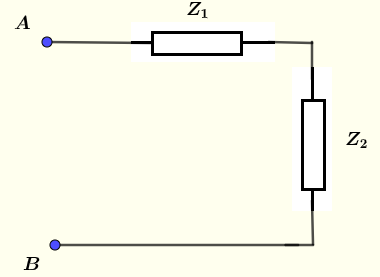The impedance of a capacitor with capacitance $C$ in complex form is equal to $\dfrac{1}{ j \omega C}$
The impedance of an inductor with inductance $L$ in complex form is equal to $j \omega L$
We now use the rule of parallel impedances to calculate $Z_2$ as
$\dfrac{1}{Z_2} = \dfrac{1}{j\omega L} + \dfrac{1}{\dfrac{1}{ j \omega C}}$
which may be written as
$\dfrac{1}{Z_2} = \dfrac{1}{j\omega L} + j \omega C$
Write the right hand side with a common denominator
$\dfrac{1}{Z_2} = \dfrac{1-\omega^2 C L}{j\omega L}$
Solve for $Z_2$
$Z_2 = \dfrac{j\omega L}{ 1-\omega^2 C L}$
Substitute $Z_1$ and $Z_2$ by their expressions to obtain $Z_{AB}$
$Z_{AB} = R + \dfrac{j\omega L}{ 1-\omega^2 C L}$
Find the modulus $|Z_{AB}|$ and argument $\theta$ of $Z_{AB}$
$|Z_{AB}| = \sqrt {R^2 + \left(\dfrac{\omega L}{ 1-\omega^2 C L )} \right)^2 }$
$\theta = \arctan \dfrac{\omega L}{R(1-\omega^2 C L)}$
In exponential form, the equivalent impedance is given by
$Z_{AB} = \sqrt {R^2 + \left(\dfrac{\omega L}{ 1-\omega^2 C L )} \right)^2 } e^{\arctan \dfrac{\omega L}{R(1-\omega^2 C L)}}$
In polar form it is written as
$Z_{AB} = \sqrt {R^2 + \left(\dfrac{\omega L}{ 1-\omega^2 C L )} \right)^2 } \; \angle \; {\arctan \dfrac{\omega L}{R(1-\omega^2 C L)}}$

Example 2
Find the equivalent impedance between points A and B in the circuit given below and write it in exponential and polar forms given:
$L_1 = 20 \; mH$ , $C_1 = 10 \; \mu F$ , $L_2 = 40 \; mH$ , $C_2 = 30 \; \mu F$ the frequency of the signal $f = 1.5 \; kHz$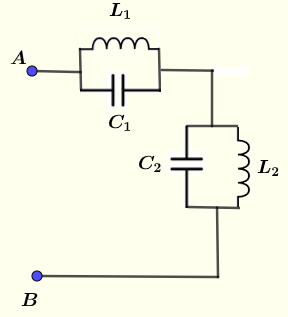Solution to Example 2
Let $Z_1$ be the impedance of the capacitor $C_1$ and the inductor $L_1$ that are in parallel.
Let $Z_2$ be the impedance of the capacitor $C_2$ and the inductor $L_2$ that are in parallel.
$Z_1$ and $Z_2$ are in series as shown below hence using We now use the rule of series impedances to calculate $Z_{AB}$ as follows
$Z_{AB} = Z_1 + Z_2$We now use the rule of parallel impedances to calculate $Z_1$ and $Z_2$ as follows
$\dfrac{1}{Z_1} = \dfrac{1}{j\omega L_1} + \dfrac{1}{\dfrac{1}{ j \omega C_1}}$
rewrite the above as
$\dfrac{1}{Z_1} = \dfrac{1}{j\omega L_1} + j \omega C_1$
write the right side with common denominator
$\dfrac{1}{Z_1} = \dfrac{1 - \omega^2 L_1 C_1}{j\omega L_1}$
Solve for $Z_1$
$Z_1 = \dfrac{j\omega L_1}{1 - \omega^2 L_1 C_1}$
$Z_2$ may be calculated in a similar way as $Z_1$ and is given by
$Z_2 = \dfrac{j\omega L_2}{1 - \omega^2 L_2 C_2}$
We now substitute $Z_1$ and $Z_2$ by their expression in $Z_{AB}$ to obtain
$Z_{AB} = \dfrac{j\omega L_1}{1 - \omega^2 L_1 C_1} + \dfrac{j\omega L_2}{1 - \omega^2 L_2 C_2}$
Fcator $j \omega$ out and rewrite $Z_{AB}$ as
$Z_{AB} = j \omega \left (\dfrac{ L_1}{1 - \omega^2 L_1 C_1} + \dfrac{ L_2}{1 - \omega^2 L_2 C_2} \right)$
Substitute by the numerical values of $L_1 , C_1 , L_2 , C_2$ and $\omega = 2 \pi f$ into $Z_{AB}$
$Z_{AB} \approx - j 14.81$
Note that $Z_{AB}$ is purte imaginary and therefore the modulus $|Z_{AB}|$ and argument $\theta$ of $Z_{AB}$ are given by
$|Z_{AB}| \approx 14.81$
$\theta = - \pi / 2$
Exponential form
$Z \approx 14.81 \; e^{-j \pi/2}$
Polar form
$Z \approx 14.81 \angle - \pi/2$

Example 3
Find the equivalent impedance between points A and B in the circuit given below and write it in exponential and polar forms given that
$R_1 = 20 \; \Omega$ , $C_1 = 50 \; \mu F$ , $C_2 = 40 \; \mu F$ , $R_2 = 80 \; \Omega$ the frequency of the signal $f = 0.5 \; kHz$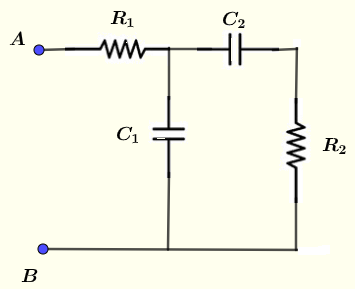Solution to Example 3
$Z_1 = R_1$
$Z_2 = \dfrac{1}{j \omega C_1}$
$Z_3 = R_2 + \dfrac{1}{j \omega C_2}$
$Z_2$ and $Z_3$ are in pararllel and their equivalent impedance $Z_{2,3}$ using the rule of parallel impedances is given by
$\dfrac{1}{Z_{2,3}} = \dfrac{1}{Z_2} + \dfrac{1}{Z_3}$
$Z_{2,3} = \dfrac{Z_2 \cdot Z_3}{Z_2 + Z_3}$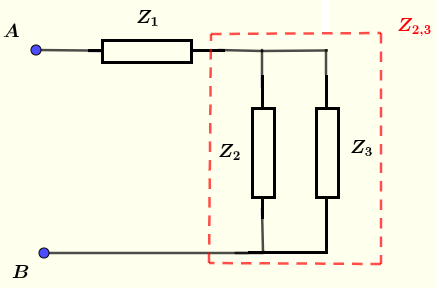$Z_1$ and $Z_{2,3}$ are in series, hence
$Z_{AB} = Z_1 + Z_{2,3} = Z_1 + \dfrac{Z_2 \cdot Z_3}{Z_2 + Z_3}$
Substitute
$Z_{AB} = R_1 + \dfrac{\dfrac{1}{j \omega C_1} \cdot (R_2 + \dfrac{1}{j \omega C_2})}{\dfrac{1}{j \omega C_1} + R_2 + \dfrac{1}{j \omega C_2}}$
Substitute by the numerical values of $R_1 = 20 \; \Omega$ , $C_1 = 50 \; \mu F$ , $C_2 = 40 \; \mu F$ , $R_2 = 80 \; \Omega$ the frequency of the signal $f = 0.5 \; kHz$ to obtain
$Z_{AB} \approx 20.49 -6.29 j$
Modulus of $Z_{AB}$
$| Z_{AB} | \approx \sqrt{20.49^2 + (-6.29)^2 } = 21.43$
Argument of $Z_{AB}$
$\theta \approx \arctan (\dfrac{-6.29}{20.49}) = -0.20 rad$ or $\theta = -17.07^{\circ}$
Hence in exponential form
$Z_{AB} \approx 21.43 e^{ -0.20 j}$
and in polar form
$Z_{AB} \approx 21.43 \angle -17.07^{\circ}$